﻿ 基于多项式的供电系统可靠性增长模型研究

# 基于多项式的供电系统可靠性增长模型研究Research on Reliability Growth Models Based on Polynomial of Power Supply System

Abstract: This paper proposes a reliability growth model and verification method based on polynomial for power system reliability. The statistical formula of the power supply reliability rate of the power supply system and the calculation formula of the customer interruption coefficient are introduced. We applying polynomial fitting modeling and fitting test method to establish a polynomial-based power supply reliability rate and customer interruption coefficient growth models for power supply system. Based on the analysis of power supply reliability statistics, the column graph is used to analyze the year-on-year change trend of power supply reliability rate for State Grid and its subordinate regional power grid city 10 kV power supply system. The calculation results of the customer interruption coefficient for State Grid and its subordinate regional power grid city 10 kV power supply system, and the pending parameter calculation and fitting test results for power supply reliability rate and customer interruption coefficient growth model are given in this paper. The results show that the power failure factor of users in urban 10 kV power supply system is decreasing, and the reliability of power supply is increasing. The variation law of power supply reliability rate and customer interruption coefficient is in accordance with the reliability growth model based on polynomial.

1. 引言

2. 供电系统可靠性的统计和评价指标

2.1. 供电可靠率的计算公式

${R}_{S1}=1-\frac{{t}_{\text{AIHC1}}}{{t}_{\text{PH}}}$ (1)

2.2. 供电系统用户停电系数的计算公式

${R}_{\text{S}1}=\frac{{t}_{\text{PH}}-{t}_{\text{AIHC1}}}{\left({t}_{\text{PH}}-{t}_{\text{AIHC1}}\right)+{t}_{\text{AIHC1}}}=\frac{1}{1+{\rho }_{\text{R1}}}$ (2)

${\rho }_{\text{R1}}=\frac{{t}_{\text{AIHC}1}}{{t}_{\text{PH}}-{t}_{\text{AIHC1}}}=\frac{1-{R}_{\text{S}1}}{{R}_{\text{S}1}}$ (3)

${\rho }_{\text{R1}}\left(t\right)=\frac{1-{R}_{\text{S1}}\left(t\right)}{{R}_{\text{S1}}\left(t\right)}$ (4)

3. 供电系统可靠性基于多项式的数学建模

3.1. 基于多项式的可靠性增长模型

$y={C}_{5}{x}^{5}+{C}_{4}{x}^{4}+{C}_{3}{x}^{3}+{C}_{2}{x}^{2}+{C}_{1}x+{C}_{0}$ (5)

$x=t$ (6)

3.2. 多项式可靠性增长模型的拟合检验方法

${R}^{2}=1-\frac{\sum {\left({y}_{i}-{y}_{j}\right)}^{2}}{\sum {\left({y}_{i}-\stackrel{¯}{y}\right)}^{2}}$ (7)

3.3. 供电可靠率基于多项式的增长模型

${R}_{\text{S1}}\left(t\right)={C}_{5}{t}^{5}+{C}_{4}{t}^{4}+{C}_{3}{t}^{3}+{C}_{2}{t}^{2}+{C}_{1}t+{C}_{0}$ (8)

3.4. 用户停电系数基于多项式的增长模型

${\rho }_{\text{R}1}\left(t\right)={c}_{5}{t}^{5}+{c}_{4}{t}^{4}+{c}_{3}{t}^{3}+{c}_{2}{t}^{2}+{c}_{1}t+{c}_{0}$ (9)

3.5. 用户停电系数为多项式的供电可靠率的计算公式

${R}_{\text{S}1}\left(t\right)=\frac{1}{1+{\rho }_{\text{R}1}\left(t\right)}=\frac{1}{1+{c}_{5}{t}^{5}+{c}_{4}{t}^{4}+{c}_{3}{t}^{3}+{c}_{2}{t}^{2}+{c}_{1}t+{c}_{0}}$ (10)

4. 全国城市10 kV用户供电可靠性为多项式增长模型的验证

4.1. 全国城市10 kV供电可靠率的统计结果Table 1. Statistical results of service reliability RS1 for customers with 10 kV of Chinese cities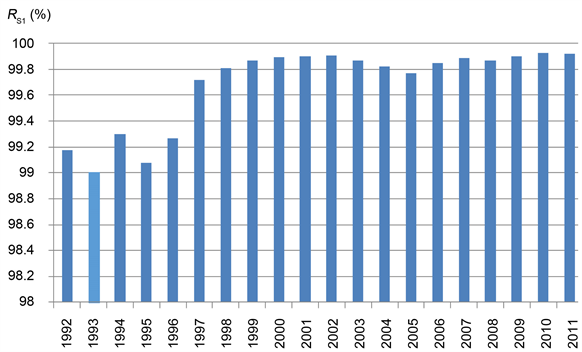Figure 1. Trend of service reliability RS1 for customers with 10 kV of Chinese cities

4.2. 全国城市10 kV供电可靠率基于多项式增长模型的验证结果

4.3. 全国城市10 kV用户停电系数的计算结果Table 2. Verification results of growth model of service reliability RS1 based on polynomial for customers with 10 kV of Chinese citiesTable 3. Calculation results of customer interruption coefficient ρR1 for power supply system with 10 kV of Chinese cities

4.4. 全国城市10 kV用户停电系数基于多项式增长模型的验证结果

5. 国家电网城市10 kV用户供电可靠性为多项式增长模型的验证

5.1. 国家电网城市10 kV供电可靠率的统计结果Table 4. Verification results of growth model of customer interruption coefficient ρR1 based on polynomial for power supply system with 10 kV of Chinese citiesTable 5. Statistical results of service reliability RS1 (%) for customers with 10 kV of State Grid cities

5.2. 国家电网城市10 kV供电可靠率基于多项式增长模型的验证结果

5.3. 国家电网城市10 kV用户停电系数的计算结果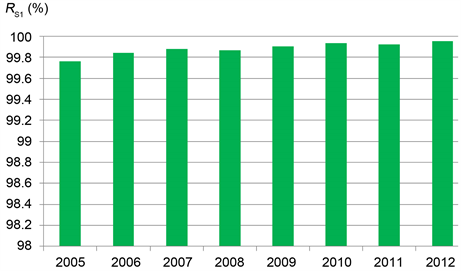Figure 2. Trend of service reliability RS1 for customers with 10 kV of State Grid cities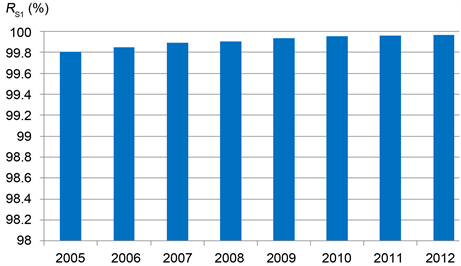Figure 3. Trend of service reliability RS1 for customers with 10 kV of North China Grid cities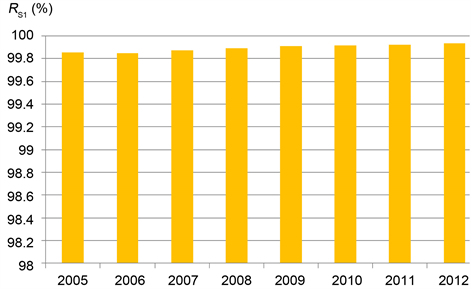Figure 4. Trend of service reliability RS1 for customers with 10 kV of Northeast China Grid cities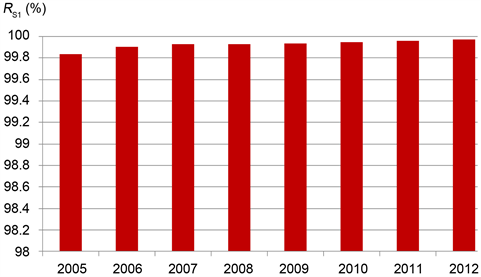Figure 5. Trend of service reliability RS1 for customers with 10 kV of East China Grid cities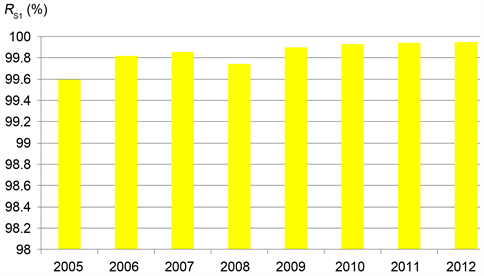Figure 6. Trend of service reliability RS1 for customers with 10 kV of Central China Grid citiesFigure 7. Trend of service reliability RS1 for customers with 10kV of Northwest China Grid citiesTable 6. Verification results of growth model of service reliability RS1 based on polynomial for customers with 10 kV of State Grid cities from 2005 to 2010Table 7. Verification results of growth model of service reliability RS1 based on polynomial for customers with 10 kV of State Grid cities from 2005 to 2012Table 8. Calculation results of customer interruption coefficient ρR1 for power supply system with 10 kV of State Grid cities

5.4. 国家电网城市10 kV用户停电系数基于多项式增长模型的验证结果Table 9. Verification results of growth model of customer interruption coefficient ρR1 based on polynomial for power supply system with 10 kV of State Grid cities from 2005 to 2010Table 10. Verification results of growth model of customer interruption coefficient ρR1 based on polynomial for power supply system with 10 kV of State Grid cities from 2005 to 2012

R2 > 0.9，表明国家电网城市10 kV供电系统的用户停电系数ρR1的变化规律符合式(9)表示的可靠性增长模型。

6. 结论

1) 采用柱形图表示的全国城市10 kV与国家电网公司及其下属区域电网的城市10 kV用户供电可靠率RS1变化趋势，表明全国城市与国家电网城市10 kV用户停电系数ρR1呈下降趋势、供电可靠率RS1呈增长趋势。

2) 全国城市10 kV与国家电网公司及其下属区域电网城市10 kV供电可靠率增长模型的定量分析和验证结果，表明供电系统的供电可靠率变化规律符合文中所建的供电可靠率基于多项式的可靠性增长模型。

3) 全国城市10 kV与国家电网公司及其下属区域电网10 kV供电系统的用户停电系数ρR1的多项式建模和拟合检验结果，表明供电系统的用户停电系数变化规律符合本文建立的用户停电系数基于多项式的增长模型。

4) 文中所建供电系统的供电可靠率RS1和用户停电系数ρR1分别为多项式的增长模型，可以表征全国城市10 kV与国家电网公司及其下属区域电网的城市10 kV用户供电系统的供电可靠性的变化趋势和可靠性增长规律。

 IEEE Transmission and Distribution Committee (1997) IEEEstd-493-1980. Recommended Practice for the Design of Reliabe Industrial and Commercial Power Systems. IEEE Standards Association, New York.

 Samotyj, M. (2006) Guideline for Reliability Assessment and Reliability Planning-Evaluation of Tools for Reliability Planning. EPRI, California.

 IEEE Transmission and Distribution Committee (2012) IEEE Std 1366TM-2012. IEEE Trial-Use Guide for Electric Power Distribution Reliability Indices. IEEE Standards Association, New York.

 电力可靠性管理中心. 中国电力可靠性管理年报[R]. 北京: 中国电力企业联合会, 2006.

 国家能源局. DL/T836-2012供电系统用户供电可靠性评价规程[S]. 北京: 中国电力出版社, 2012.

 中华人民共和国国家质量监督检验检疫总局, 中国国家标准化管理委员会. GB/T2900.13-2008/IEC60050(191): 1990. 电工术语可信性与服务质量[S]. 北京: 中国标准出版社, 2009.

 同济大学数学教研室. 线性代数(第三版) [M]. 北京: 高等教育出版社, 1999.

 方开泰, 全辉, 陈庆云. 实用回归分析[M]. 北京: 科学出版社, 1988: 22-151.

Top